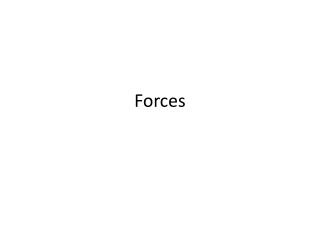Download PresentationForces

# Forces - PowerPoint PPT PresentationDownload Presentation## Forces

- - - - - - - - - - - - - - - - - - - - - - - - - - - E N D - - - - - - - - - - - - - - - - - - - - - - - - - - -
##### Presentation Transcript

1. Forces

2. SPECIFIC LEARNING OUTCOMES: • By the end of this topic you should be able to: • Give examples of everyday forces (push/pull/twist) • Label simple force diagrams (driving/thrust, reaction/support/buoyancy, friction/drag, gravity) • Recognise the distance forces of gravity and magnetism and the contact force of friction and be able to apply their knowledge to contexts as given • Use arrows as indicator of force size and direction • Label a simple net force diagram, and calculate net forces • demonstrate/describe the effect of net force (collisions) • describe the difference between weight (a force due to gravity) and mass (the amount of matter in an object) • stopping distances / reaction times • understand how simple machines work (levers or pulleys or…) • Motion (optional) • calculate speed • interpret speed-time graphs • interpret distance-time graph

3. A force is a push or a pull • For example the following forces are: • Gravity Push / pull (Circle correct) • A strong wind blowing • a car over. Push / pull • Removing bluetack • from the wall with • your fingers. Push / pull • Advanced examples for experts… • A piston in a car. Push / pull • Air passing over an • Airplane wing. Push / pull • Forces have direction. • We use arrows to show the direction that the force is acting. Example: • Direction of force is indicated by the arrow.

4. Forces Motion and Velocity • In Science we like to use the word velocity instead of the word speed. This is because velocity is a measure of speed and direction. Speed does not normally include the direction in which an object was traveling. For example • A train is traveling at a speed of 68 km hr-1 • A train is traveling north west at a velocity of 68 km hr-1

5. How to calculate velocity • The formula for velocity is   Velocity (v) = distance (d) time (t) d v t

6. Distance Vs Time Graphs • Distance time graphs clearly show the motion (movement) of an object. • More slope (steeper) means the velocity of the object is ________. • Less slope (flatter) means the velocity of the object is ________. • If the line is completely flat then the object is ________. • Time • Distance Vs Time • Distance • On the graph above – label where: • The object is stationary • The object has the greatest velocity • The object is moving backwards -

7. Distance vs time Graphs

8. Copy the graph above and label where: • The object is stationary • The object has the greatest velocity • The object is moving backwards -

9. The faster you go the bigger the mess

10. Mass and Weight • It's important to understand the difference between weight and mass. • We often use the terms "mass" and "weight" interchangeably in our daily speech, but in their true meanings they are completely different things. • The mass of a object is how much matter (atoms) it contains. • Your weight is the force on the ground caused by gravity.

11. To measure your weight all you have to do is stand on a scale! Scales measure the force of attraction between you and the Earth. This force of attraction between you and the Earth (or any other planet) is called your weight. • If you are in a spaceship far between the stars and you put a scale underneath you, the scale would read zero. Your weight is zero. You are weightless. There is an anvil floating next to you. It's also weightless. But are you or the anvil mass-less?.... No.